Rhythm Chains

+ Associated example files

NB: An exercise relating to the material covered in this tutorial can be found on the Exercises page.

The rthm-chain class provides a means of algorithmically generating two-voice rthm-seq-palettes and rthm-seq-maps that can be inserted directly into a slippery-chicken object. It creates the two voices by automatically assembling sequences of user-defined rhythmic fragments of either one, two, or three beats.

One of the class's more versatile features is its diversification of the user-defined source material through the automatic insertion of rests and the automatic repetition of what it calls sticking rhythms at certain points in the new material. Both of these features can be influenced by the user through the specification of patterns for the insertion.

The class also offers the user arguments that control the level of activity in the two voices (more notes vs. more rests) by means of envelopes.

+ The "procession" algorithm

The primary sequence-generating function at the core of the rthm-chain class is the procession algorithm. This function is used by default to internally determine the resulting order of the user-defined fragments in the resulting palettes and maps by extrapolating a list of a specified length from items of a specified starting list.

Using procession with a list

The procession algorithm is purely deterministic; i.e., it incorporates no randomness. It starts with the first three elements of the initial list and gradually adds successive elements from that list until all of the elements have been added. The initial list must therefore have at least four elements.

(procession 73 '(a b c d e))

=>
(A B A B C A C A A D B C B D B C B C D A D A A E C D C E B D B D E C D C C
E A B A E B D B D E A C A A E B D B E C D C D E A B A A E C D C E B D B)

Using procession with an integer

Instead of passing a list to the function as its second argument, the user can instead pass an integer. In this case, the function will generate its output from a list of consecutive integers from 1 to the integer passed as the second argument.

(procession 73 4)

=>
(1 2 1 2 3 1 3 1 1 4 2 3 2 4 2 3 2 3 4 1 3 1 1 4 2 3 2 4 1 3 1 3 4 2 3 2 2
4 1 3 1 4 2 3 2 3 4 1 2 1 1 4 2 3 2 4 1 3 1 3 4 2 3 2 2 4 1 3 1 4 1 3 1)

close

+ The procession keyword arguments

The procession function has three optional arguments that contribute to determining the sequence that is generated, namely :peak, :expt, and :orders.

:peak

The value passed to the :peak argument has an influence on the position at which the last item of the original list first appears in the new list. It takes a floating-point number between 0.0 and 1.0 as its value, representing a span of 0% to 100% of the way through the new list, and it defaults to 0.7.

It is important to note that this value is an approximate value only. Shorter initial lists will reflect this percentage value less accurately, and the position of the final item in the resulting list will also be dependent on other factors, such as the values of the :orders argument discussed below.

:expt

The value passed to the :expt argument determines the curve of the interval at which each subsequent element of the original list is added to the new list. This too is an approximate argument, and its accuracy will again be influenced by aspects such as the lengths of the original and resulting lists.

In general, the higher this number is, the steeper the exponential curve will be. A steeper curve means that subsequent elements of the original list will be introduced less frequently towards the beginning of the new list, and then more frequently towards the end. This argument also takes a floating-point number, and it defaults to 1.3.

:orders

This argument takes a list of sublists that represent the patterns by which elements from the original list are introduced to the list returned by the procession function.

Each consists of all of and only the numbers 1, 2, and 3. These numbers represent the three least used elements in the new list at each pass through the function's list-generating loop. The patterns are cyclically applied and default to '((1 2 1 2 3) (1 2 1 1 3) (1 2 1 3)).

The following is an example of the use of the procession algorithm using all three of these keywords:

(procession 500 '(a b c d e f g h i j k l m n o p q r s t u v w x y z)
:peak 0.9
:expt 0.7
:orders '((1 3 2 2 3 1) (3 1 1 2 3 3) (1 2 1 3 2 2)))

=> (A C B B C A E A A D E E B D B F D D C F E E F C G A A F G G B C B G C C D H
F F H D H E E G H H G H G I H H A I B B I A J D D I J J F I F J I I C J E E
J C K G G J K K I J I K J J A K B B K A L D D K L L F K F L K K C L H H L C
M E E L M M G L G M L L A M I I M A N B B M N N D M D N M M F N H H N F O J
J N O O K N K O N N L O M M O L P N N O P P C O C P O O E P O O P E P G G I
P P A P A Q P P B Q D D Q B Q F F H Q Q I J I Q J J K R Q Q R K R L L Q R R
M Q M R Q Q N S R R S N S O O R S S P R P S R R C S R R S C T E E S T T G S
G T S S H T S S T H T A A Q T T B T B U T T D U T T U D U F F I U U K L K U
L L M V U U V M V N N U V V O U O V U U P V U U V P W Q Q V W W R V R W V V
S W V V W S W T T V W W C E C W E E G X W W X G X H H W X X I W I X W W J X
U U X J Y V V X Y Y A X A Y X X B Y X X Y B Y D D X Y Y F X F Y X X K Z Y Y
Z K Z M M Y Z Z N Y N Z Y Y O Z P P Z O Z Q Q R Z Z S T S Z T T W Z Y Y Z W
Z C C G Z Z H I H J I I L U R R U L Z A A V Z Z B D B E D D F J G G J F N K
K M N N O P)

close

+ General attributes of the make-rthm-chain function

A rthm-seq-map and a rthm-seq-palette

The user can create a new object of the rthm-chain class using the function make-rthm-chain. The rthm-chain class is a subclass of the rthm-seq-map class, one of whose slots is designed to contain a rthm-seq-palette. The make-rthm-chain function therefore creates an object that defines a rthm-seq-map and automatically generates an accompanying rthm-seq-palette for that map. This palette can be accessed using (palette rthm-chain-object).

The map itself and the contents of the map's palette slot can both be passed directly to the :rthm-seq-map and :rthm-seq-palette arguments of the make-slippery-chicken function, as described below.

Internal implementation of the procession algorithm

By default, the make-rthm-chain function will use the procession algorithm to generate its sequences. The user must therefore define at least four 1-beat and four 2- and 3-beat rhythm fragments within the corresponding arguments of the function call (see below). Due to the way these rhythms are processed and assembled, the rhythm fragments defined must not contain any individual rhythms that overlap beats.

Keyword arguments

The make-rthm-chain function has 17 optional keyword arguments. These influence traits such as the activity levels of the two voices (rests vs. notes), the automatic rest insertion, and the automatic insertion of "sticking rhythms", as described below.

close

+ Explaining make-rthm-chain through a simple example

The following simple example demonstrates the argument requirements, syntax, and usage of the make-rthm-chain function.

(let* ((rch
(make-rthm-chain
'test-rch 143
'((((e) e) ; 4 in total
(- s (s) (s) s -)
({ 3 (te) - te te - })
((e.) s))
(({ 3 (te) te (te) }) ; what we transition to
({ 3 - te (te) te - })
({ 3 (te) - te te - })
({ 3 (te) (te) te }))
(((q)) ; the second transition
(- s e s -)
({ 3 te (tq) })
(s (e.)))
((- e e -) ; the third transition
(- s s s - (s))
((32) 32 (e.))
((q))))
'((((q q) ; the 2/4 bars: 4 total
((q) q)
((q) q)
((q) (s) e.))
(({ 3 te+te te+te te+te }) ; what we transition to
(q - s e. -)
(q (s) e.)
(q (s) - s e -))
((q - e e -) ; the second transition
((e) q.)
(q - s (e) s -)
((s) q e.)))
((((e.) s (e) e (s) e.) ; the 3/4 bars: 4 total
(- e e - (e) e (q))
(- e. s - - +e e - (q))
(q (e.) s (q)))
(({ 3 (te) (te) te+te te+te } (q)) ; what we transition to
(- e. s - (q) (s) - s e -)
({ 3 te+te te } (q) q)
({ 3 - te te te - } (e) e { 3 (te) (te) te }))
((h.) ; the second transition
(h (q))
(- e e - +q (q))
(s (e.) (h)))))
:players '(fl cl)))
(mini
(progn
(create-psps (palette rch))
(make-slippery-chicken
'+mini+
:ensemble '(((fl (flute :midi-channel 1))
(cl (b-flat-clarinet :midi-channel 2))))
:set-palette '((1 ((e2 a2 cs4 fs4 gs4 a4 b4 e5 gs5 b5 e6))))
:set-map `((1 ,(ml 1 (num-rthm-seqs rch))))
:tempo-map '((1 (q 120)))
:rthm-seq-palette (palette rch)
:rthm-seq-map rch))))
(midi-play mini)
(cmn-display mini)
(write-lp-data-for-all mini))

The first two arguments passed to the make-rthm-chain function consist of a user-defined ID for the rthm-chain object being created and an integer that determines the length of the resulting musical passage, in the form of the number of bars, that the function is to generate. Remember that these bars will only be one, two, or three beats long.

The user-defined source rhythm fragments

1-beat fragments

The next two arguments are the lists of source fragments defined by the user. The first list must consist of 1-beat fragments, and there must be at least four of these. These rhythms will be used to create the first voice of the resulting object, and can be thought of as the fast-moving voice.

'((((e) e) ; 4 in total
(- s (s) (s) s -)
({ 3 (te) - te te - })
((e.) s)))
2- and 3-beat fragments

The next argument to the make-rthm-chain function takes a list of two sublists. The first of these sublists takes at least four 2-beat rhythmic fragments, and the second takes at least four 3-beat rhythmic fragments.

These fragments will form the second voice of the resulting object, and can be thought of as the slower-moving counterpoint (though the user can, of course, also fill these lists with fast rhythms if preferred). Remember that the rhythms defined here must not overlap beats. If longer durations are desired, the beats should be divided up and connected using ties.

'((((q q) ; the 2/4 bars: 4 total
((q) q)
((q) q)
((q) (s) e.)))
((((e.) s (e) e (s) e.) ; the 3/4 bars: 4 total
(- e e - (e) e (q))
(- e. s - - +e e - (q))
(q (e.) s (q)))))

player IDs

Although the :players argument is technically optional, the user will generally want to specify the player IDs in the call to make-rthm-chain for best results. These IDs must be the same as those specified in the ensemble of the slippery-chicken object in which this rthm-chain object will be used.

:players '(fl cl))))
(let* ((mini
(make-slippery-chicken
'+mini+
:ensemble '(((fl (flute :midi-channel 1))
(cl (b-flat-clarinet :midi-channel 2))))

Automatic pitch-seqs

The object created by make-rthm-chain will contain a rthm-seq-palette with individual rthm-seq objects, but the pitch-seq-palettes of those rthm-seqs will be initially empty. A convenient and quick way of creating pitch-seqs for the pitchless rhythms in this, or indeed any, rthm-seq-palette is the create-psps method.

The create-psps method must be called separately, after the creation of the rthm-seq-palette object for which it is to create pitch-seqs. It takes one argument, namely the rthm-seq-palette that it is to handle, which in this case is accessed using the "palette" slot accessor, as described above.

The pitch-seqs generated by this method are created from combinations of a list of default pitch-seqs if not otherwise specified. The user can, however, modify these lists to shape the resulting pitch curves to his or her own taste. See the documentation for create-psps for more detail.

(create-psps (palette rch))

Implementing the resulting palette and map

The resulting palette and map can then be passed directly to the :rthm-seq-palette and :rthm-seq-map arguments of the make-slippery-chicken function, as seen here:

:rthm-seq-palette (palette rch)
:rthm-seq-map rch)))

The first system of the piece created by the above code will look like this in LilyPond output: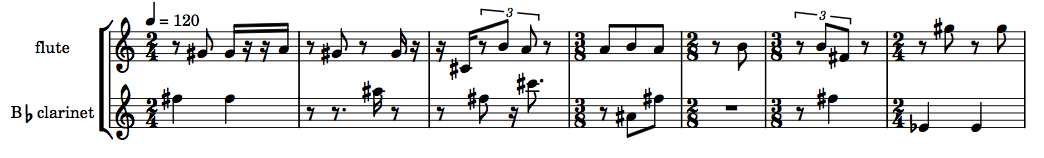close

+ Using fibonacci-transitions instead of procession

The user also has the option of using the fibonacci-transitions algorithm instead of the procession algorithm to generate the resulting sequences. In order for the make-rthm-chain function to know to apply the fibonacci-transitions algorithm instead, the user must set one or both of the keyword arguments :1-beat-fibonacci (the 1-beat fragments) and :slow-fibonacci (the 2- and 3-beat fragments) to T.

:1-beat-fibonacci t
:slow-fibonacci t

The rest remains the same

All other aspects of usage in conjunction with the fibonacci-transition function remain the same, e.g.:

(let* ((rch
(make-rthm-chain
'test-rch 143
'((((e) e) ; 4 in total
(- s (s) (s) s -)
({ 3 (te) - te te - })
((e.) s)))
'((((q q) ; the 2/4 bars: 4 total
((q) q)
((q) q)
((q) (s) e.)))
((((e.) s (e) e (s) e.) ; the 3/4 bars: 4 total
(- e e - (e) e (q))
(- e. s - - +e e - (q))
(q (e.) s (q)))))
:players '(fl cl)
:1-beat-fibonacci t
:slow-fibonacci t)))
(create-psps (palette rch))
(let* ((mini
(make-slippery-chicken
'+mini+
:ensemble '(((fl (flute :midi-channel 1))
(cl (b-flat-clarinet :midi-channel 2))))
:set-palette '((1 ((e2 a2 cs4 fs4 gs4 a4 b4 e5 gs5 b5 e6))))
:set-map `((1 ,(ml 1 (num-rthm-seqs rch))))
:tempo-map '((1 (q 120)))
:rthm-seq-palette (palette rch)
:rthm-seq-map rch)))
(midi-play mini)
(cmn-display mini)
(write-lp-data-for-all mini)))

The following is the LilyPond output of the same first system as the first example, this time using fibonacci-transitions for both the 1-beat and the slow voices: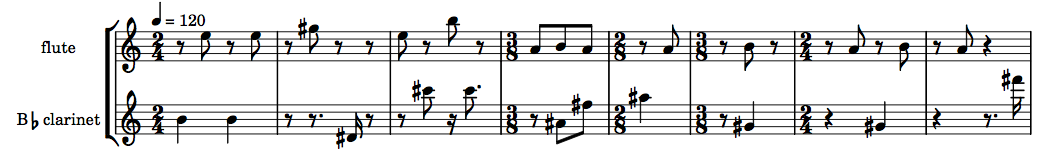close

+ An extra level of fibonacci-transitions

The make-rthm-chain function has an additional feature that allows the user to specify a further level of fibonacci-transitioning in the musical data that it generates. By specifying one or more additional sublists of fragments, the function will automatically perform a transition from the elements of the initial sets to the elements of each consecutive set.

This approach requires that each subsequent sublist have the same number of elements. It also requires that both the 2-beat and 3-beat fragments defined have the same number of additional sublists (the number of 1-beat sublists can differ from that of the 2- and 3-beat sublists). This approach can be used with both the procession and the fibonacci-transitions algorithms as the initial sequence generators.

(let* ((rch
(make-rthm-chain
'test-rch 143
'((((e) e) ; 4 in total
(- s (s) (s) s -)
({ 3 (te) - te te - })
((e.) s))
(({ 3 (te) te (te) }) ; what we transition to
({ 3 - te (te) te - })
({ 3 (te) - te te - })
({ 3 (te) (te) te }))
(((q)) ; the second transition
(- s e s -)
({ 3 te (tq) })
(s (e.)))
((- e e -) ; the third transition
(- s s s - (s))
((32) 32 (e.))
((q))))
'((((q q) ; the 2/4 bars: 4 total
((q) q)
((q) q)
((q) (s) e.))
(({ 3 te+te te+te te+te }) ; what we transition to
(q - s e. -)
(q (s) e.)
(q (s) - s e -))
((q - e e -) ; the second transition
((e) q.)
(q - s (e) s -)
((s) q e.)))
((((e.) s (e) e (s) e.) ; the 3/4 bars: 4 total
(- e e - (e) e (q))
(- e. s - - +e e - (q))
(q (e.) s (q)))
(({ 3 (te) (te) te+te te+te } (q)) ; what we transition to
(- e. s - (q) (s) - s e -)
({ 3 te+te te } (q) q)
({ 3 - te te te - } (e) e { 3 (te) (te) te }))
((h.) ; the second transition
(h (q))
(- e e - +q (q))
(s (e.) (h)))))
:players '(fl cl))))
(create-psps (palette rch))
(let* ((mini
(make-slippery-chicken
'+mini+
:ensemble '(((fl (flute :midi-channel 1))
(cl (b-flat-clarinet :midi-channel 2))))
:set-palette '((1 ((e2 a2 cs4 fs4 gs4 a4 b4 e5 gs5 b5 e6))))
:set-map `((1 ,(ml 1 (num-rthm-seqs rch))))
:tempo-map '((1 (q 120)))
:rthm-seq-palette (palette rch)
:rthm-seq-map rch)))
(midi-play mini)
(cmn-display mini)
(write-lp-data-for-all mini)))

The following two snippets show the difference in bars 23 to 38 between the first procession example, and the code above.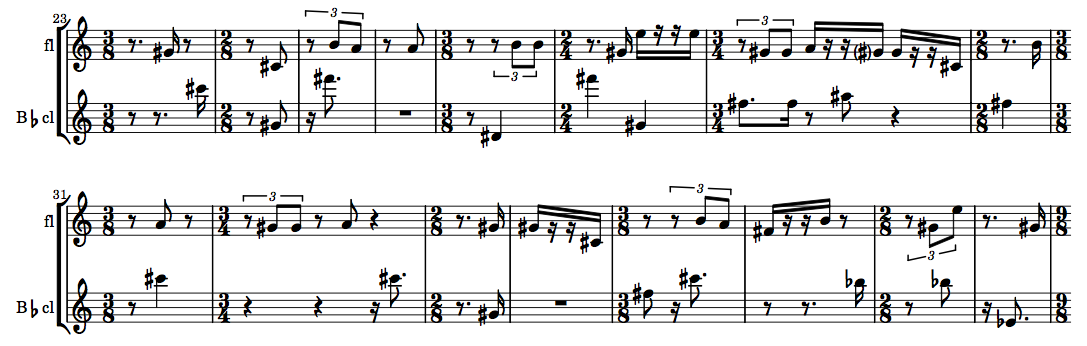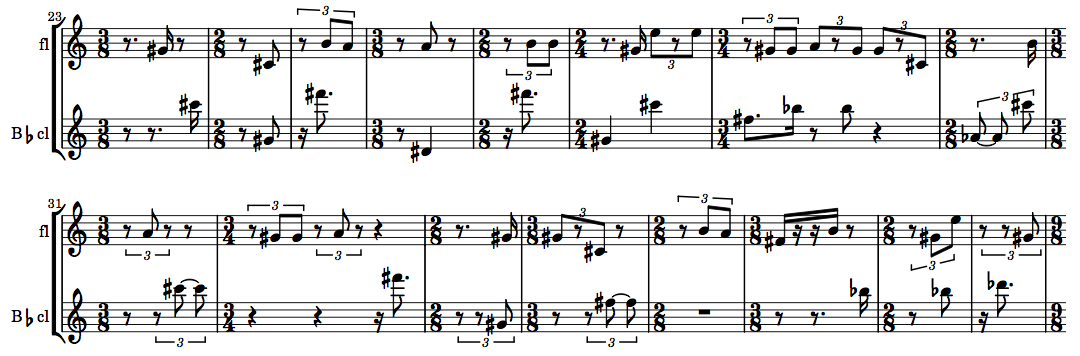close

+ Some useful post-generation editing methods

Because of the fragmented nature of the source material for this featured algorithm, the resulting music may often be the point of departure for slightly more extensive use of post-generation editing methods. For example, the user may want to consolidate tied notes or multiple consecutive rests, add ties between existing notes or extend short notes over subsequent rests, or consolidate the very short bars into longer ones for easier reading. A number of methods are available to assist the user to these ends.

close

+ consolidate-notes

The consolidate-notes method combines any tied notes of a specified bar into one longer duration. This is a complex algorithm and is not fool-proof; for example, it does not split new durations evenly at the beats of the bar. But it can still be a very valuable aid in assembling a more legible score.

This method can be particularly useful with rthm-chain objects that were created from 2- and 3-beat fragments containing ties between beats, as can be seen in the following before-and-after snippets of the same passage.The consolidate-notes method takes a single rthm-seq-bar object as its only argument. When applied to an existing slippery-chicken object, the bar object is best specified using the get-bar method, which takes the slippery-chicken object, the bar number, and the player ID as its own arguments, as can be seen here:

(consolidate-notes (get-bar mini 72 'cl))

This method is often automatically called from within other methods. In such cases, it can usually be disabled by setting the :consolidate-notes keyword argument to NIL, if the user so desires.

close

+ consolidate-rests-max

This method performs a similar consolidation routine to that of consolidate-notes, but applied to rests instead. As with the note-consolidating algorithm, this algorithm too is quite complex and not fool-proof, with the similar caveat that the resulting, longer rests are not split evenly at beats.

The result of calling this method on bar 2 of the flute part in the second rthm-chain object created above can be seen in these before-and-after snippets of the score:As with the consolidate-notes method, this method takes a single rthm-seq-bar object as its only argument. This too can best be retrieved using the same get-bar syntax spelled out above:

(consolidate-rests-max (get-bar mini 2 'fl))

This method too is built-in to many other methods, and can generally be disabled in those methods by specifying the :consolidate-rests keyword with the value NIL.

close

+ tie-over-notes

One of the rthm-chain class's primary features, the automatic insertion of rests of various durations, may result in music that is initially a little pointillistic. One option for smoothing out passages of jagged short notes is the use of the tie-over-rests and related methods.

The tie-over-rests method changes all consecutive rests immediately following a specified note into notes of the same pitch as the note specified and ties them all together. It takes as its arguments the slippery-chicken object to be modified, the number of the bar in which the tie is to begin, the number of the note from which the tie is to begin, and the player ID.

(tie-over-rests mini 72 4 'fl)

This brief before-and-after example demonstrates the effect of using tie-over-rests on some of the notes from the original object.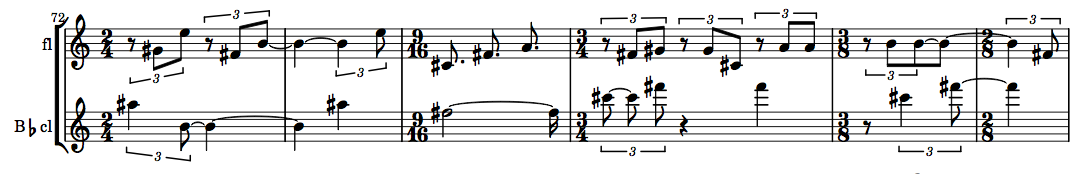By default, the tie-over-rests method also applies the consolidate-notes algorithm automatically to the bars affected. This behavior can be disabled by setting the method's :consolidate-notes keyword argument to NIL, e.g.:

(tie-over-rests mini 72 4 'fl :consolidate-notes nil)

It is important to note that this method changes the number of notes (non-rest event objects) in the specified bar and potentially any subsequent bars that begin with rests. Because of this, it may be necessary, when applying the method several times in the same player of a piece, to apply it in backwards order and set the :consolidate-notes argument to NIL.

It is also important to note that this method only affects the printable output. In order for any new ties to also be reflected in the MIDI output, the user must subsequently call the handle-ties method for the entire slippery-chicken object.

(handle-ties mini)

close

+ map-over-bars

Many of the post-generation editing methods only apply to one bar at a time. If the user would like to apply such a method to a longer passage of consecutive measures, the method map-over-bars can be very helpful.

This method takes as its arguments the slippery-chicken object to be affected, the start bar, end bar, and player ID (or list of IDs) for the part(s) to be affected, the method (or function) that is to be applied, and any additional optional arguments which that method or function may possess.

(map-over-bars mini 72 77 'cl #'consolidate-notes)

The result of this call to map-over-bars with the same passage used in the consolidate-notes example above can be seen in the score snippets here: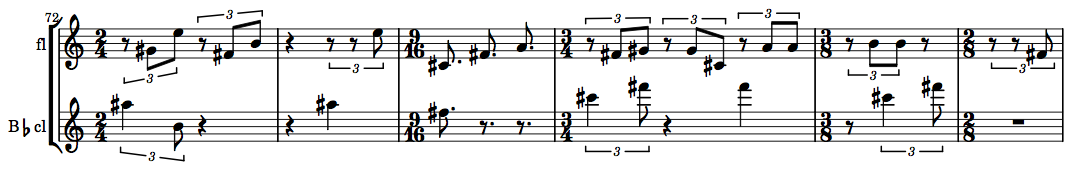close

+ re-bar

The re-bar method is handy for redistributing musical material consisting of several short bars into fewer, longer bars. It is discussed in more detail in the tutorial for Second Law and Intraphrasal-Looping, but will be reviewed briefly here as well.

The re-bar method will only combine shorter bars into longer ones; it will not divide longer bars into shorter bars in order to conform to a strict time signature. In its simplest form, it takes as its arguments the slippery-chicken object to be re-barred, and a time signature passed to the min-time-sig keyword argument, which serves as the target time signature of for the re-barring process.

(re-bar mini :min-time-sig '(4 4))

It is important to note that because the method functions by merely combining consecutive bars, it will not always be possible to produce bars of the exact time signature given here (depending on the time signatures of the original bars), and the user should consider the value passed to this argument to be a generally descriptive target rather than a precise value.

The following two score snippets provide a before-and-after view of applying the re-bar method to the first rthm-chain object above.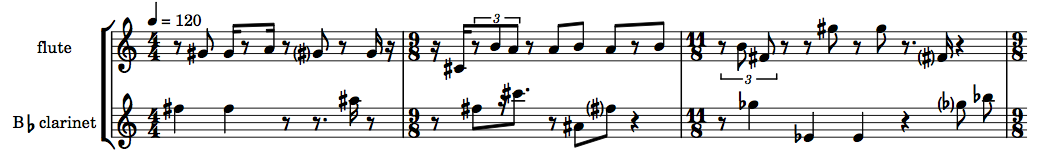close

The final rthm-chain method to be discussed here is add-voice. This method allows the user to automatically generate a new voice for the same original two-voice rthm-seq-palette and rthm-seq-map using the same fragment material specified in the initial call to make-rthm-chain.

When calling add-voice, the user must specify the rthm-chain object that is to be extended, the ID of the existing player from whose part the new voice is to be derived (coupled with the section ID of the rthm-chain object's rthm-seq-map, which defaults to 1), and the player ID for the new part, e.g.:

The add-voice method must be called before create-psps is called, if the user intends to automatically generate pitch-seq-palettes for all parts, e.g.:

(create-psps (palette rch))

If the rthm-chain object generated is to be used immediately in a call to make-slippery-chicken (as will usually be the case), the :ensemble defined for that function must also include a player with the same ID specified in the call to add-voice, e.g.:

:ensemble '(((fl (flute :midi-channel 1))
(ob (oboe :midi-channel 2))
(cl (b-flat-clarinet :midi-channel 3))))

The method generates its new part from the original part by first analyzing the time signatures of the rthm-seq objects of that source part. It collects the sequences of that first part into groups of same meter in the order they occur, and creates a new voice from different rthm-seqs of identical meter to the source voice.

The following is a small amount of source code and an accompanying score fragment of the music generated by that code produced by LilyPond:

(let* ((rch
(make-rthm-chain
'test-rch 173
'((((e) e) ; 4 in total
(- s (s) (s) s -)
({ 3 (te) - te te - })
((e.) s)))
'((((q q) ; the 2/4 bars: 4 total
((q) q)
((q) q)
((q) (s) e.)))
((((e.) s (e) e (s) e.) ; the 3/4 bars: 4 total
(- e e - (e) e (q))
(- e. s - - +e e - (q))
(q (e.) s (q)))))
:players '(fl cl))))
(create-psps (palette rch))
(let* ((mini
(make-slippery-chicken
'+mini+
:ensemble '(((fl (flute :midi-channel 1))
(ob (oboe :midi-channel 2))
(cl (b-flat-clarinet :midi-channel 3))))
:set-limits-high '((fl (0 e6 100 e6))
(ob (0 b5 100 b5))
(cl (0 d5 100 d5)))
:set-limits-low '((fl (0 gs5 100 gs5))
(ob (0 a4 100 a4))
(cl (0 e3 100 e3)))
:tempo-map '((1 (q 72)))
:set-palette '((1 ((cs4 fs4 gs4 a4 b4 e5 gs5 b5 e6))))
:set-map `((1 ,(ml 1 (num-rthm-seqs rch))))
:rthm-seq-palette (palette rch)
:rthm-seq-map rch)))
(midi-play mini)
(cmn-display mini)
(write-lp-data-for-all mini)))close